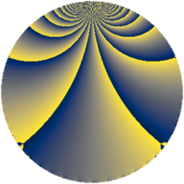Properties

 Label 1728.1.gLevel $1728$ Weight $1$ Character orbit 1728.g Rep. character $\chi_{1728}(703,\cdot)$ Character field $\Q$ Dimension $2$ Newform subspaces $1$ Sturm bound $288$ Trace bound $0$

Related objects

Defining parameters

 Level: $$N$$ $$=$$ $$1728 = 2^{6} \cdot 3^{3}$$ Weight: $$k$$ $$=$$ $$1$$ Character orbit: $$[\chi]$$ $$=$$ 1728.g (of order $$2$$ and degree $$1$$) Character conductor: $$\operatorname{cond}(\chi)$$ $$=$$ $$4$$ Character field: $$\Q$$ Newform subspaces: $$1$$ Sturm bound: $$288$$ Trace bound: $$0$$

Dimensions

The following table gives the dimensions of various subspaces of $$M_{1}(1728, [\chi])$$.

Total New Old
Modular forms 52 2 50
Cusp forms 16 2 14
Eisenstein series 36 0 36

The following table gives the dimensions of subspaces with specified projective image type.

$$D_n$$ $$A_4$$ $$S_4$$ $$A_5$$
Dimension 2 0 0 0

Trace form

 $$2 q + O(q^{10})$$ $$2 q - 2 q^{13} - 2 q^{25} + 2 q^{37} - 4 q^{49} + 2 q^{61} + 2 q^{73} + 2 q^{97} + O(q^{100})$$

Decomposition of $$S_{1}^{\mathrm{new}}(1728, [\chi])$$ into newform subspaces

Label Dim. $$A$$ Field Image CM RM Traces $q$-expansion
$a_{2}$ $a_{3}$ $a_{5}$ $a_{7}$
1728.1.g.a $2$ $0.862$ $$\Q(\sqrt{-3})$$ $D_{6}$ $$\Q(\sqrt{-3})$$ None $$0$$ $$0$$ $$0$$ $$0$$ $$q+(\zeta_{6}+\zeta_{6}^{2})q^{7}-q^{13}+(\zeta_{6}+\zeta_{6}^{2}+\cdots)q^{19}+\cdots$$

Decomposition of $$S_{1}^{\mathrm{old}}(1728, [\chi])$$ into lower level spaces

$$S_{1}^{\mathrm{old}}(1728, [\chi]) \cong$$ $$S_{1}^{\mathrm{new}}(144, [\chi])$$$$^{\oplus 6}$$$$\oplus$$$$S_{1}^{\mathrm{new}}(432, [\chi])$$$$^{\oplus 3}$$$$\oplus$$$$S_{1}^{\mathrm{new}}(576, [\chi])$$$$^{\oplus 2}$$